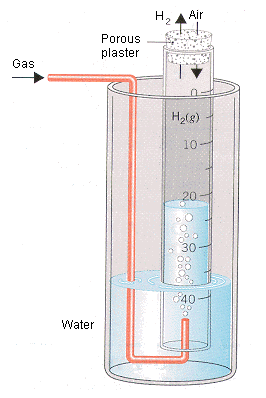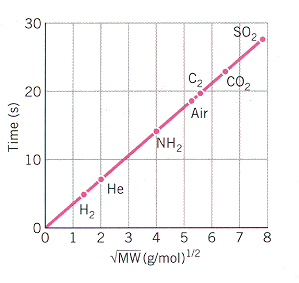Thomas Graham

Graham's Laws of Diffusion and Effusion (Thomas Graham)

A few of the physical properties of gases depend on the identity of the gas. One of these physical properties can be seen when the movement of gases is studied.

In 1829 Thomas Graham used an apparatus similar to the one shown in Figure 4.15 to study the diffusion of gases -- the rate at which two gases mix. This apparatus consists of a glass tube sealed at one end with plaster that has holes large enough to allow a gas to enter or leave the tube. When the tube is filled with H2 gas, the level of water in the tube slowly rises because the H2 molecules inside the tube escape through the holes in the plaster more rapidly than the molecules in air can enter the tube. By studying the rate at which the water level in this apparatus changed, Graham was able to obtain data on the rate at which different gases mixed with air.The rate of diffusion, or mixing of a gas with air, can be studied with the apparatus shown here.  If the gas escapes from the tube faster than the air enters the tube, the amount of water in the tube will increase.  If air enters the tube faster than the gas escapes, water will be displaced from the tube.

Graham found that the rates at which gases diffuse is inversely proportional to the square root of their densities.

This relationship eventually became known as Graham's law of diffusion.

To understand the importance of this discovery we have to remember that equal volumes of different gases contain the same number of particles. As a result, the number of moles of gas per liter at a given temperature and pressure is constant, which means that the density of a gas is directly proportional to its molecular weight. Graham's law of diffusion can therefore also be written as follows.

Similar results were obtained when Graham studied the rate of effusion of a gas, which is the rate at which the gas escapes through a pinhole into a vacuum. The rate of effusion of a gas is also inversely proportional to the square root of either the density or the molecular weight of the gas.

Graham's law of effusion can be demonstrated with the apparatus shown below. A thick-walled filter flask is evacuated with a vacuum pump. A syringe is filled with 25 mL of gas and the time required for the gas to escape through the syringe needle into the evacuated filter flask is measured with a stop watch. The experimental data in the table below were obtained by using a special needle with a very small (0.015 cm) hole through which the gas could escape.The rate of effusion of a gas can be demonstrated with the apparatus shown here.   The time recquired for the gas in the syringe to escape into an evacuated flask is measured.  The faster the gas molecules effuse, the less time it takes for a given volume of the gas to escape into the flask

The Time Required for 25-mL Samples of Different Gases to Escape through a 0.015 cm Hole into a Vacuum

 Compound Time (s) Molecular Weight H2 5.1 2.02 He 7.2 4.00 NH3 14.2 17.0 air 18.2 29.0 O2 19.2 32.0 CO2 22.5 44.0 SO2 27.4 64.1

As we can see when these data are graphed below, the time required for 25-mL samples of different gases to escape into a vacuum is proportional to the square root of the molecular weight of the gas. The rate at which the gases effuse is therefore inversely proportional to the square root of the molecular weight. Graham's observations about the rate at which gases diffuse (mix) or effuse (escape through a pinhole) suggest that relatively light gas particles such as H2 molecules or He atoms move faster than relatively heavy gas particles such as CO2 or SO2 molecules.A graph of the time required for 25-mL samples of different gases to escape into an evacuated flask versus the square root of the molecular weight of the gas.   Relatively heavy molecules move more slowly, and it takes more time for the gas to escape.

The Kinetic Molecular Theory and Graham's Laws (Thomas Graham)

The kinetic molecular theory can be used to explain the results Graham obtained when he studied the diffusion and effusion of gases. The key to this explanation is the last postulate of the kinetic theory, which assumes that the temperature of a system is proportional to the average kinetic energy of its particles and nothing else. In other words, the temperature of a system increases if and only if there is an increase in the average kinetic energy of its particles.

Two gases, such as H2 and O2, at the same temperature, therefore, must have the same average kinetic energy. This can be represented by the following equation.

This equation can be simplified by multiplying both sides by two.

It can then be rearranged to give the following.

Taking the square root of both sides of this equation gives a relationship between the ratio of the velocities at which the two gases move and the square root of the ratio of their molecular weights.

This equation is a modified form of Graham's law. It suggests that the velocity (or rate) at which gas molecules move is inversely proportional to the square root of their molecular weights.